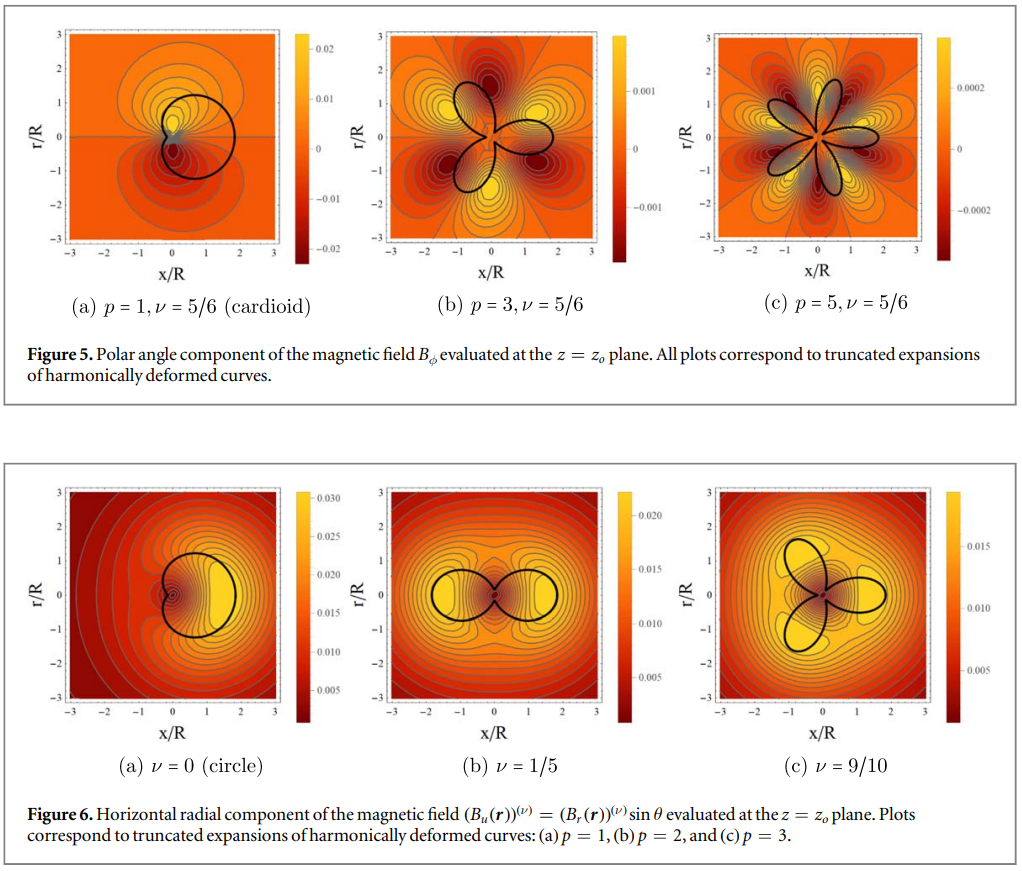## Expansion formula for the magnetic field of a periodically deformed circular current loop

#### Physica Scripta 96, 125502 (2021)

A method is derived to obtain an expansion formula for the magnetic field B(r) generated by a closed planar wire carrying a steady electric current. The parametric equation of the loop is R(ϕ)=R+Hf(ϕ), with R the radius of the circle, H∈[0,R) the radial deformation amplitude, and f(ϕ)∈[−1,1] a periodic function. The method is based on the replacement of the 1/|r−r′|3 factor by an infinite series in terms of Gegenbauer polynomials, as well as the use of the Taylor series. This approach makes it feasible to write B(r) as the circular loop magnetic field contribution plus a sum of powers of H/R. Analytic formulas for the magnetic field are obtained from truncated finite expansions outside the neighborhood of the wire. These showed to be computationally less expensive than numerical integration in regions where the dipole approximation is not enough to describe the field properly. Illustrative examples of the magnetic field due to circular wires deformed harmonically are developed in the article, obtaining exact expansion coefficients and accurate descriptions. Error estimates are calculated to identify the regions in R3 where the analytical expansions perform well. Finally, first-order deformation formulas for the magnetic field are studied for generic even deformation functions f(ϕ).

`Universidad de los Andes | Vigilada MineducaciónReconocimiento como Universidad: Decreto 1297 del 30 de mayo de 1964.Reconocimiento personería jurídica: Resolución 28 del 23 de febrero de 1949 Minjusticia.`